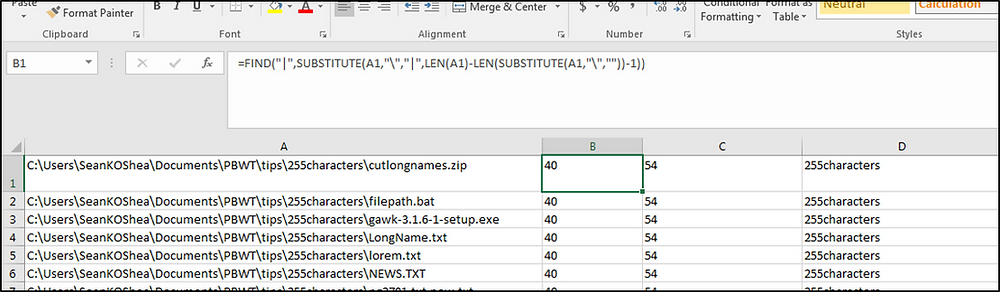Search

# Excel Formula to Extract Last Subfolder in a File Path

Here's a series of Excel formulas which will allow you to extract the last subfolder in a file path. Starting with the file path in column A enter the following formulas in columns B, C, and D:

=FIND("|",SUBSTITUTE(A1,"\","|",LEN(A1)-LEN(SUBSTITUTE(A1,"\",""))-1))

=FIND("\",A1,B1+1)

=MID(A1,B1+1,(C1-B1)-1)

The first formula indicates how many characters into column A the next to last backward slash is. The second formula indicates the position in the file path that the last backward slash appears at. The last formula find the text between the beginning and ending points listed in columns B and C.Thanks to Todd for posting this solution at http://superuser.com/questions/969898/split-folder-paths-in-excel-to-return-the-final-folder